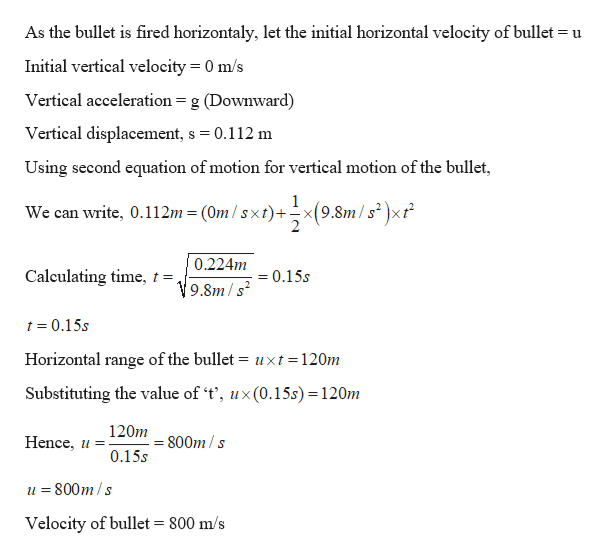# A rifle is aimed horizonally toward the center of a target 120m away. If the bullet strikes 11.2cm below the center, what was the velocity of the bullet?(ignore air friction)

Question
36 views

A rifle is aimed horizonally toward the center of a target 120m away. If the bullet strikes 11.2cm below the center, what was the velocity of the bullet?(ignore air friction)

check_circle

Step 1

Given:

Horizontal distance covered by bullet = 120 m

Vertical distance covered by bullet = 11.2 cm = 0.112 m

Step 2

Calculating the initial vel...help_outlineImage TranscriptioncloseAs the bullet is fired horizontaly, let the initial horizontal velocity of bullet = u Initial vertical velocity 0 m/s Vertical acceleration = g (Downward) Vertical displacement, s = 0.112 m Using second equation of motion for vertical motion of the bullet, 9.8m/s2x We can write, 0.112m = (0m /sxt)+ 0.224m Calculating time, t =, =0.15s 9.8m/s2 t 0.15s Horizontal range of the bullet = uxt=120m Substituting the value of 't', ux(0.15s) 120m 120m Hence, u = 800m/s 0.15s 800m/s Velocity of bullet = 800 m/s fullscreen

### Want to see the full answer?

See Solution

#### Want to see this answer and more?

Solutions are written by subject experts who are available 24/7. Questions are typically answered within 1 hour.*

See Solution
*Response times may vary by subject and question.
Tagged in

### Kinematics